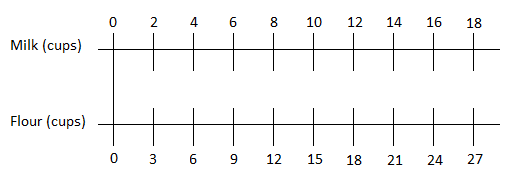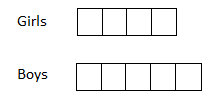# Unit Rates and Percent

Students investigate rates and percentages by identifying the rates associated with a ratio, defining a percent as a rate per 100, and applying strategies to solve rate and percent problems.

## Unit Summary

In Unit 2, sixth grade students continue and extend their study of ratios to investigate rates and percentages. Using their knowledge of ratios, students will learn to identify two rates associated with a ratio and use them as efficient strategies to solve rate problems. Students will draw on their prior knowledge of the two measurement systems, and see unit conversions as applications of ratio and rate problems. Lastly, students will define a percent as a rate per 100, and understand that the strategies you use to solve a rate problem can also be applied to solve a percent problem. Throughout the entire unit, students will draw on their ability to reason abstractly and quantitatively (MP.2). As they work with different unit rates and conversion factors, students will need to attend to the units at hand, what the quantities mean, and how all of the pieces fit together. Calculators are recommended for several lessons in order to provide students with the option to use the tool in their calculations (MP.5).

In fourth and fifth grade, students interpreted fractions as division problems and began to make the connection between fractions and decimals. They multiplied fractions by whole numbers and other fractions in context of real-world problems, and they reasoned about what happens to a quantity when you multiply it by a number greater than one or less than one. Sixth grade students will draw on these prior skills and understandings as they make connections between unit rates and fractions, and between fractions, decimals, and percentages.

Beyond this unit, sixth grade students will revisit percentages in Unit 6 when they study equations as another strategy to solve percent problems. In seventh grade, students will solve even more complex ratio, rate, and percent problems involving, for example, tax and percent increase or decrease. Seventh graders will investigate and analyze proportional relationships between quantities, and use more efficient and abstract methods to solve problems. For example, in seventh grade, students will learn to set up and solve proportion equations, using their knowledge of unit rates and equivalent ratios. (Note that using proportions to solve ratio or rate problems is not an expectation in sixth grade).

Pacing: 19 instructional days (14 lessons, 4 flex/review days, 1 assessment day)

For guidance on adjusting the pacing for the 2021-2022 school year, see our 6th Grade Scope and Sequence Recommended Adjustments.• Unit Launch
• Expanded Assessment Package
• Problem Sets for Each Lesson
• Student Handout Editor
• Vocabulary Package

## Assessment

This assessment accompanies Unit 2 and should be given on the suggested assessment day or after completing the unit.

## Unit Prep

### Intellectual Prep

?

#### Internalization of Standards via the Post-Unit Assessment

• Take the Post-Unit Assessment. Annotate for:
• Standards that each question aligns to
• Strategies and representations used in daily lessons
• Relationship to Essential Understandings of unit
• Lesson(s) that Assessment points to

#### Internalization of Trajectory of Unit

• Read and annotate the Unit Summary.
• Notice the progression of concepts through the unit using the Lesson Map.
• Essential Understandings
• Connection to Post-Unit Assessment questions
• Identify key opportunities to engage students in academic discourse. Read through our Teacher Tool on Academic Discourse and refer back to it throughout the unit.

#### Unit-Specific Intellectual Prep

Model Example
Double number line For every 2 cups of milk, there are 3 cups of flour.Ratio table A turtle travels 3 feet every 9 seconds.
 Distance (ft) Time(sec) 3 9 6 18 9 27 30 90
Tape diagram The ratio of girls to boys in a 6th grade class is 4 to 5.### Essential Understandings

?

• A rate, associated with a ratio a:b, is a/b or b/a units of one quantity per 1 unit of the other quantity. For example, if a person walks 6 miles in 2 hours, the person is traveling at a rate of 3 miles per hour, or equivalently a rate of 1/3 hour per mile.
• A percent is a rate per 100; percent problems can be solved using the same strategies that are used for other rate problems.
• There are many applications to rate, including unit price, constant speed, and measurement conversions, that can be solved using several different strategies, such as using double number lines, tables, tape diagrams, and the unit rate.

### Vocabulary

?

unit rate

conversion rate

customary system

metric system

rate

ratio

equivalent ratio

percent/ percentage

To see all the vocabulary for this course, view our 6th Grade Vocabulary Glossary.

?

# 1

6.RP.A.3

6.RP.A.3.B

Investigate and use rate in real-world situations.

# 2

6.RP.A.2

6.RP.A.3.B

Define rate and unit rate, and find rates from situations involving ratios.

# 3

6.RP.A.2

6.RP.A.3.B

Find unit rates and use them to solve problems.

# 4

6.RP.A.3.B

Compare situations using unit rates, including speed, price, and work problems.

# 5

6.RP.A.2

6.RP.A.3

6.RP.A.3.B

Solve challenging problems involving unit rate.

# 6

6.RP.A.3.D

Reason with measurement units and solve unit conversion problems using different strategies.

# 7

6.RP.A.3.D

Solve measurement unit conversion problems.

# 8

6.RP.A.3.C

Define percent as a rate per 100. Find percentages of 100 and 1.

# 9

6.RP.A.3.C

Solve percent problems using benchmark fractions and percentages, with and without written work.

# 10

6.RP.A.3.C

Convert between fractions, percent, and decimals.

# 11

6.RP.A.3.C

Find a percent of a quantity.

# 12

6.RP.A.3.C

Find the whole quantity, given a part and percent.

# 13

6.RP.A.3.C

Solve percent problems, including finding percent when given a part and a whole.

# 14

6.RP.A.2

6.RP.A.3

6.RP.A.3.C

6.RP.A.3.D

Solve multi-step problems involving rate and percent.

## Common Core Standards

Key: Major Cluster Supporting Cluster Additional Cluster

### Core Standards

?

##### Ratios and Proportional Relationships
• 6.RP.A.2 — Understand the concept of a unit rate a/b associated with a ratio a:b with b ≠ 0, and use rate language in the context of a ratio relationship. Expectations for unit rates in this grade are limited to non-complex fractions. For example, "This recipe has a ratio of 3 cups of flour to 4 cups of sugar, so there is 3/4 cup of flour for each cup of sugar." "We paid $75 for 15 hamburgers, which is a rate of$5 per hamburger."

• 6.RP.A.3 — Use ratio and rate reasoning to solve real-world and mathematical problems, e.g., by reasoning about tables of equivalent ratios, tape diagrams, double number line diagrams, or equations.

• 6.RP.A.3.B — Solve unit rate problems including those involving unit pricing and constant speed. For example, if it took 7 hours to mow 4 lawns, then at that rate, how many lawns could be mowed in 35 hours? At what rate were lawns being mowed?

• 6.RP.A.3.C — Find a percent of a quantity as a rate per 100 (e.g., 30% of a quantity means 30/100 times the quantity); solve problems involving finding the whole, given a part and the percent.

• 6.RP.A.3.D — Use ratio reasoning to convert measurement units; manipulate and transform units appropriately when multiplying or dividing quantities.

?

• 4.MD.A.1

• 4.MD.A.2

• 5.MD.A.1

• 5.NBT.B.6

• 4.NF.B.4.B

• 4.NF.B.4.C

• 4.NF.C.6

• 5.NF.B.3

• 5.NF.B.4.A

• 5.NF.B.5

• 5.NF.B.5.A

• 5.NF.B.5.B

• 5.NF.B.6

• 6.RP.A.1

?

• 6.EE.B.7

• 6.EE.C.9

• 7.RP.A.1

• 7.RP.A.2

• 7.RP.A.3

### Standards for Mathematical Practice

• CCSS.MATH.PRACTICE.MP1 — Make sense of problems and persevere in solving them.

• CCSS.MATH.PRACTICE.MP2 — Reason abstractly and quantitatively.

• CCSS.MATH.PRACTICE.MP3 — Construct viable arguments and critique the reasoning of others.

• CCSS.MATH.PRACTICE.MP4 — Model with mathematics.

• CCSS.MATH.PRACTICE.MP5 — Use appropriate tools strategically.

• CCSS.MATH.PRACTICE.MP6 — Attend to precision.

• CCSS.MATH.PRACTICE.MP7 — Look for and make use of structure.

• CCSS.MATH.PRACTICE.MP8 — Look for and express regularity in repeated reasoning.